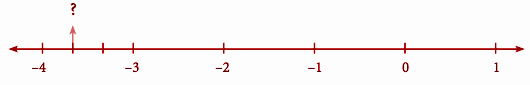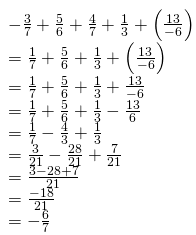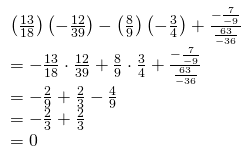Samacheer Kalvi 8th Maths Book Solutions Term 1 Chapter 1 – Rational Numbers are available here. The Samacheer Kalvi 8th Maths book answers of Term 1 Chapter 1, available at BYJU’S, contain step by step explanations designed by our mathematics experts. All these important questions are based on the new pattern prescribed by the Tamil Nadu board. Students can also get the solutions of other chapters on Samacheer Kalvi 8th Maths solutions. This Samacheer Kalvi Class 8 Maths Book Term 1 Chapter 1 Questions and Solutions is a useful resource for students to practice and thoroughly gauge their knowledge of a topic.

## Samacheer Kalvi 8th Class Maths Textbook Term 1 Chapter 1 Questions with Solutions

Term 1 Chapter 1 of the Samacheer Kalvi 8th Maths guide will help the students to solve problems related to rational numbers, rational numbers on a number line, writing a decimal as a rational number, equivalent rational numbers, rational numbers in standard form, comparison of rational numbers, rational numbers between any two given rational numbers, basic operations on rational numbers, properties for rational numbers.

### Samacheer Kalvi 8th Maths Term 1 Chapter 1: Rational Numbers Book Exercise 1.1 Questions and Solutions

Question 1: List five rational numbers between -2 and 0.

Solution:

-2 and 0

-2 / 1 and 0 / 1

(-2 / 1) = (-2 * 10) / (1 * 10) = -20 / 10

(0 / 1) = (0 * 10) / (1 * 10) = 0 / 10

The five rational numbers between -2 and 0 are (-20 / 10), (-19 / 10), (-18 / 10), (-7 / 10), (-6 / 10), (-5 / 10), (0 / 10).

Question 2: Find the rational numbers for the question marks marked on the number line.Solution:

The number with the question mark lies between -3 and -4. The part between -3 and -4 is divided into 3 equal parts and the second part is the question. The number is -3 (2 / 3) = (-11 / 3).

Question 3: Rearrange suitably and add: -3 / 7 + 5 / 6 + 4 / 7 + 1 / 3 + (13 / -6).

Solution:### Samacheer Kalvi 8th Maths Chapter 1: Rational Numbers Book Exercise 1.2 Questions and Solutions

Question 1: Verify the closure property for addition and multiplication of the rational numbers −5 / 7 and 8 / 9.

Solution:

a = -5 / 7 and b = 8 / 9

a + b = (-5 / 7) + (8 / 9)

= [-45 + 56] / 63

= 11 / 63

Closure property is true for addition of rational numbers.

Closure property of multiplication

a = -5 / 7 and b = 8 / 9

a * b = (-5 / 7) * (8 / 9)

a * b = -40 / 63

Closure property is true for multiplication of rational numbers.

Question 2: Verify the associative property for addition and multiplication of the rational

numbers (-10 / 11), 5 / 6, (-4 / 3).

Solution:

a = (-10 / 11), b = 5 / 6, c = (-4 / 3)

(a + b) + c = a + (b + c)

(a + b) + c

= (-10 / 11 + 5 / 6) +(-4 / 3)

= (-5 / 66) + (-4 / 3)

= -93 / 66

= -31 / 22 — (1)

a + (b + c)

= -10 / 11 + (5 / 6 +(-4 / 3))

= (-10 / 11) + (-3 / 6)

= -93 / 66

= -31 / 22 — (2)

From (1) and (2), (a + b) + c = a + (b + c).

(a * b) * c = a * (b * c)

(a * b) * c

= [(-10 / 11) * (5 / 6)] * (-4 / 3)

= (-50 / 66) * (-4 / 3)

= 100 / 99 — (1)

a * (b * c)

= (-10 / 11) * [(5 / 6) * (-4 / 3)]

= (-10 / 11) * (-20 / 18)

= 100 / 99 — (2)

From (1) and (2), (a * b) * c = a * (b * c)

Question 3: Evaluate (13 / 18) (-12 / 39) – (8 / 9) (-3 / 4) + (-7 / -9) ÷ (63 / -36).

Solution:### Samacheer Kalvi 8th Maths Term 1 Chapter 1: Rational Numbers Book Exercise 1.3 Questions and Solutions

Question 1: Subbu spends 1 / 3 of his monthly earnings on rent, 2 / 5 on food and 1 / 10 on monthly usuals. What fractional part of his earnings is left with him for other expenses?

Solution:

Total spending made by Subbu = (1 / 3) + (2 / 5) + (1 / 10)

= (10 + 12 + 3) / 30

= 25 / 30

= 5 / 6

If the total earning is Re.1, then total spending is 5 / 6.

Fraction of money left with Subbu = (1 – [5 / 6]) = 1 / 6

Question 2: In recipe making, every 1 (1 / 2) cup of rice requires 2 (3 / 4) cups of water. Express this, in the ratio of rice to water.

Solution:

Rice required = 1 (1 / 2) cup

Water required = 2 (3 / 4) cup

Rice / Water = [1 (1 / 2)] / [2 (3 / 4)]

= (3 / 2) * (4 / 11)

= 6 / 11

Rice: Water = 6:11

Question 3: A piece of wire is 4 / 5 m long. If it is cut into 8 pieces of equal length, how long will each piece be?

Solution:

Length of the wire = (4 / 5) m = (4 * 100) / 5 = 400 / 5 = 80cm

Number of pieces made = 8

Length of a single piece = 80 / 8 = 10cm

Hence, the length of each piece is 10cm.